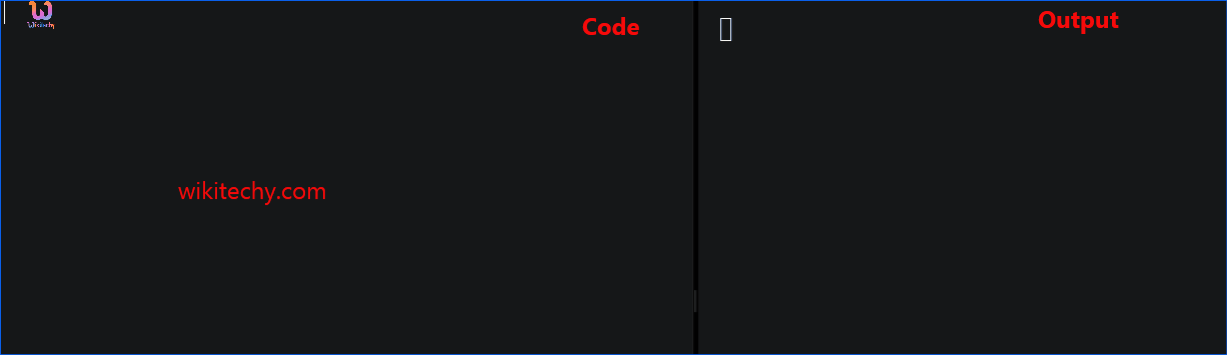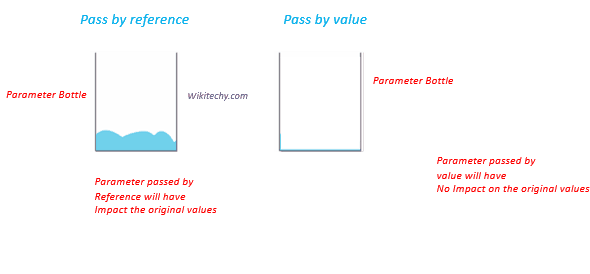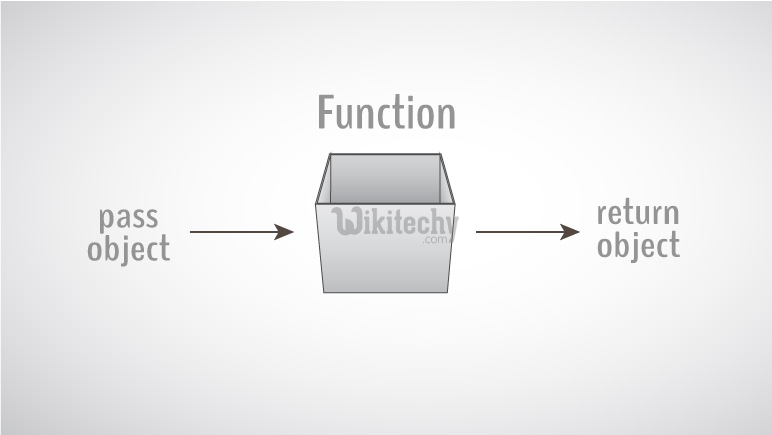# Structure and Function | C++ Structure and Function - Learn C++ - C++ Tutorial - C++ programmingLearn c++ - c++ tutorial - c++-structure-and-function - c++ examples - c++ programs

## Structure And Function in C++

• Structure is a collection of variables under a single variable.
• Structure variables can be passed to a function and returned in a similar way as normal argument.
• A function is a group of statements that together perform a task.
•learn c++ tutorials - structure and functions Example

• Every C++ program has at least one function, which is main(), and all the most trivial programs can define additional functions. You can divide up your code into separate functions.

## Passing structure to function in C++• A structure variable can be passed to a function in similar way as normal argument.
• When an element of a structure is passed to a function, you are actually passing the values of that element to the function. Therefore, it is just like passing a simple variable (unless, of course, that element is complex such as an array of character). Consider this example:

## Output

• In this program, user is asked to enter the name, age and salary of a Person inside main() function.
• Then, the structure variable p is to passed to a function using.
• The return type of displayData() is void and a single argument of type structure Person is passed.
• Then the members of structure p are displayed from this function.